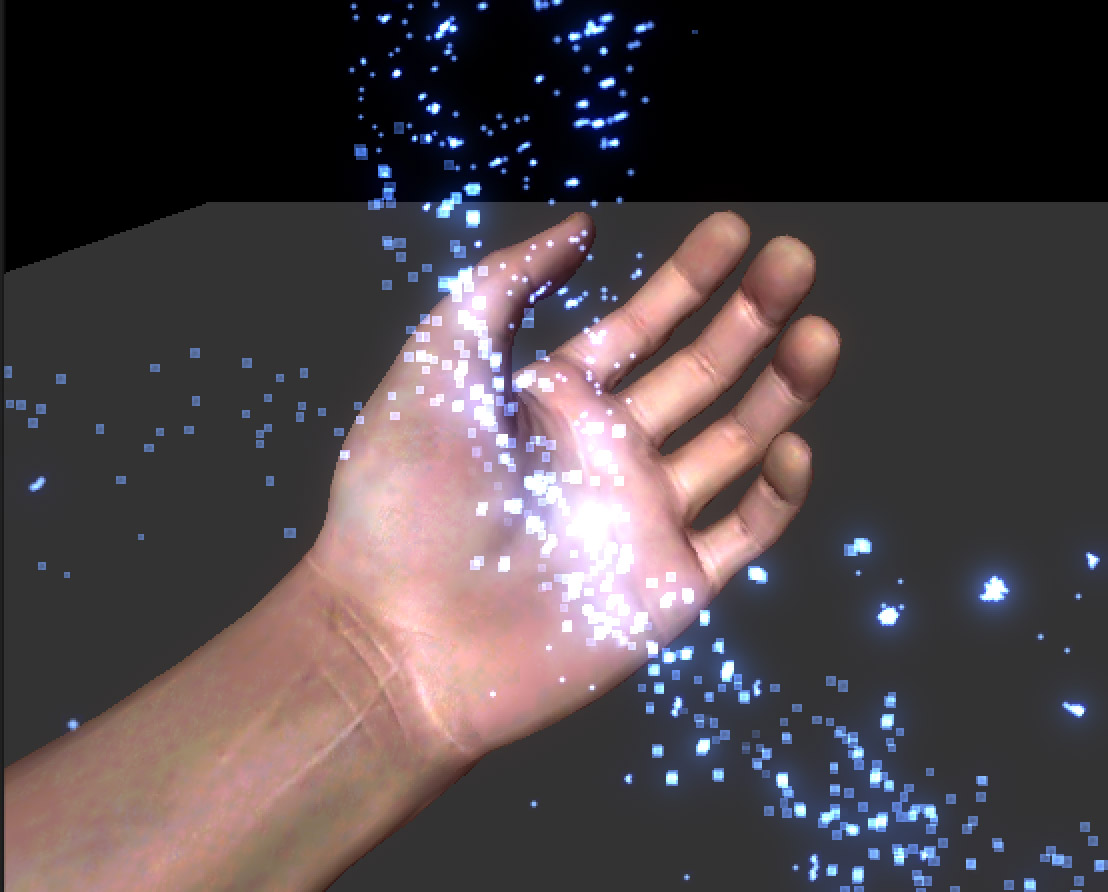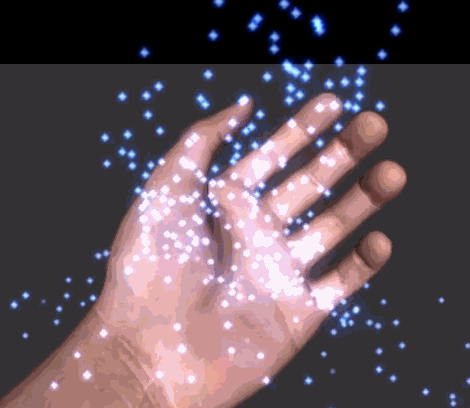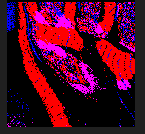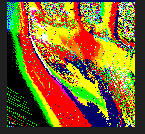# Jim's GameDev Blog# 基于 GPU 的粒子碰撞（实践篇）

2016-7-2

Screenshot GIF// vertex shader
float2 bias = v.color.rg * 2 - 1;
float4 vert = v.vertex;
vert.xyz = 0;
float4 wPos = mul(_Object2World, vert);
wPos.xyz += normalize(UNITY_MATRIX_V.xyz) * bias.r * _SizeW + normalize(UNITY_MATRIX_V.xyz) * bias.g * _SizeH;
o.vertex = mul(UNITY_MATRIX_VP, wPos);
o.uv = TRANSFORM_TEX(v.uv, _MainTex);


// vertex shader
float2 bias = v.color.rg * 2 - 1;
float4 vert = v.vertex;
// 每个粒子的坐标不会重叠，我们利用 tangent.zw 来表示各个粒子之间的间隔
vert.xyz = float3(v.tangent.z, 0, v.tangent.w);
float4 wPos = mul(_Object2World, vert);
wPos.xyz += normalize(UNITY_MATRIX_V.xyz) * bias.r * _SizeW + normalize(UNITY_MATRIX_V.xyz) * bias.g * _SizeH;

// 原本在这里将时间代入轨迹公式中，用公式返回的结果对顶点位置进行偏移。
// 但是我们不这么做。
// 取而代之的是，从一张纹理中取出当前顶点对应的像素点的值，这个像素点的值就是当前顶点的坐标偏移量。

o.vertex = mul(UNITY_MATRIX_VP, wPos);
o.uv = TRANSFORM_TEX(v.uv, _MainTex);


// vertex shader
float2 bias = v.color.rg * 2 - 1;
float4 vert = v.vertex;
vert.xyz = float3(v.tangent.z, 0, v.tangent.w);
float4 wPos = mul(_Object2World, vert);
wPos.xyz += normalize(UNITY_MATRIX_V.xyz) * bias.r * _SizeW + normalize(UNITY_MATRIX_V.xyz) * bias.g * _SizeH;

// _positionsRT 就是记录每个粒子位置的纹理（RenderTexture）
// tangent.xy 记录了从纹理的哪个像素中读取数据
// _rtTexelSize 是纹理尺寸的倒数，表示单个像素占多少个 uv 值
float4 texWPos = tex2D(_positionsRT, v.tangent.xy*_rtTexelSize);
wPos.xyz += texWPos.xyz;

o.vertex = mul(UNITY_MATRIX_VP, wPos);
o.uv = TRANSFORM_TEX(v.uv, _MainTex);// 设置所需的所有 uniform 值
// 这些值在向 RenderTexture 填充数据时会用到
// 设置输出的 RenderTexture
// 注意，我把输出和输入的 RenderTexture 设置成了同一个，这在某些设备上可能会出现问题
// 为了简化问题暂且这么做，在最后会再次提到这个问题
fxCamera.targetTexture = positionsRT;
// 这里我使用 fxCamera 来专门渲染粒子，你也可以通过修改 mainCamera 的 culllayers 来完成



// vertex shader
float4 vert = v.vertex;

vert.xyz = float3(v.tangent.z, 0, v.tangent.w);
// 取出粒子的坐标
float4 texWPos = tex2D(_positionsRT, v.tangent.xy*_rtTexelSize);
// 取出粒子的速度（这个会在后面讲到，是另一张纹理用来存储粒子的速度）

float4 texWVel = tex2D(_velocitiesRT, v.tangent.xy*_rtTexelSize) * 2 - 1;
float4 wPos = mul(_Object2World, vert);
// 判断粒子是否已经在地面以下了

bool underGround = (wPos + texWPos).y < -30;
// 这一步非常关键
// 由于是从 v.tangent.xy 这个 uv 坐标中取出的值，所以还要将计算后的值写回这个 uv 坐标下的 texel
// 如果写到其他粒子所使用的 texel，就会影响到其他粒子的运动了
o.vertex = float4(v.tangent.xy*_rtTexelSize * 2.0 - 1.0 + v.color.rg * _rtTexelSize*2, 0.5f, 1);
// 如果在地面以下，将坐标重置到起始点
if(underGround)

{
o.texWPos = 0;
}
else
{
// 坐标加上速度，即为下一帧时的坐标点
// 这里对坐标点的计算很简单，你也可以使用更复杂的非匀速运动方程
o.texWPos = texWPos + texWVel * 0.1;
}
return i.texWPos;// 手动调用计算粒子速度的着色器代码
fxCamera.targetTexture = velocitiesRT;


float4 vert = v.vertex;

vert.xyz = float3(v.tangent.z, 0, v.tangent.w);
float4 texWPos = tex2D(_positionsRT, v.tangent.xy*_rtTexelSize);

float4 texWVel = tex2D(_velocitiesRT, v.tangent.xy*_rtTexelSize) * 2 - 1;
// 在粒子速度上加上一个向下的重力速度

texWVel += float4(0, -0.08, 0, 0);
float4 wPos = mul(_Object2World, vert);

bool underGround = (wPos + texWPos).y < -30;
o.vertex = float4(v.tangent.xy*_rtTexelSize * 2.0 - 1.0 + v.color.rg * _rtTexelSize*2, 0.5f, 1);

if(underGround)

{
o.texWVel = float4(0, 0, 0.5, 0);
}
else
{
o.texWVel = (texWVel + 1) * 0.5;
}
// 下面开始计算碰撞到物体产生反弹的效果
float depth;
float3 normal;
float4 projPos2 = mul(_fxCamVP, mul(_Object2World, vertV) + float4(texWPos.xyz, 0));
float2 projUV2 = (projPos2.xy / projPos2.w + 1) * 0.5;
// 现在将其中的法线和深度信息取出来

// 计算当前粒子的深度值
float thisDepth = -mul(_fxCamV, mul(_Object2World, vertV) + float4(texWPos.xyz, 0)).z / _fxCamFar;
// 通过对比两个深度值，判断当前粒子是否被场景中的物体挡住
if(thisDepth > depth)
{
normal = mul((float3x3)_fxCamV2W, normal);
normal = normalize(normal);
// 如果挡住了，对速度向量以法线为轴心进行反射
float3 vel = reflect(o.texWVel, normal);
// 将新的速度写回到 _velocitiesRT 中
o.texWVel.xyz = vel;
}